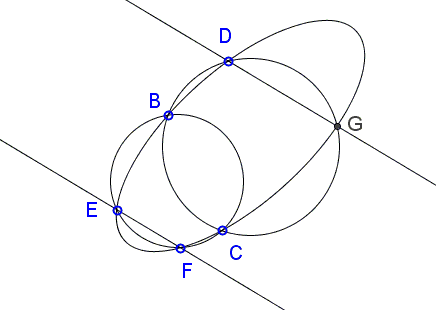# Two Circles, Ellipse, and Parallel Lines

The problem below has been posted at the CutTheKnotMath facebook page by Cõ Gẫng Lên:

Given circles $C_{CBEF}$ through $B, C, E, F$, $C_{BCGD}$ through $B,C,D,G$, and ellipse $E_{BEFCGD}$ through all six points.Prove that the lines $DG$ and $EF$ are parallel.

SolutionGiven circles $C_{CBEF}$ through $B, C, E, F$, $C_{BCGD}$ through $B,C,D,G$, and ellipse $E_{BEFCGD}$ through all six points.Prove that the lines $DG$ and $EF$ are parallel.

I shall assume that $C_{XYZW}$ also denotes the quadratic expression such that $C_{XYZW} = 0$ is the equation of circle $C_{XYZW}$ through points $X,Y,Z,W,$ and similarly for ellipses. In particular, we are given circles $C_{CBEF}=0,$ $C_{BCGD}=0,$ and ellipse $E_{BEFCGD}=0.$ In addition, a line through points $X,Y$ will be denoted $L_{XY}$ and defined by the equation $L_{XY}=0.$

The pencil of conics through four points $B, C, E, F$

$\lambda C_{CBEF} + \mu E_{BEFCGD} = 0$

contains a degenerate conic $L_{BC}\cdot L_{EF} = 0,$ of two (intersecting or parallel) lines $L_{BC}=0$ and $L_{EF}=0,$ meaning that, for some fixed $\lambda_1$ and $\mu_1,$

$L_{BC}\cdot L_{EF} = \lambda_{1} C_{CBEF} + \mu_{1} E_{BEFCGD}.$

Both $L_{BC}=0$ and $L_{EF}=0$ are linear equations.

Similarly, for some fixed $\lambda_2$ and $\mu_2,$

$L_{BC}\cdot L_{DG} = \lambda_{2} C_{BCGD} + \mu_{2} E_{BEFCGD}.$

Combining the two equations we obtain

$L_{BC}\cdot (\mu_{2}L_{EF} - \mu_{1}L_{DG}) = \lambda_{1}\mu_{2}C_{CBEF} - \lambda_{2}\mu_{1}C_{BCGD}.$

Now, on one hand, equation $\lambda_{1}\mu_{2}C_{CBEF} - \lambda_{2}\mu_{1}C_{BCGD} = 0$ defines a member of a pencil of circles through points $B,C.$ This pencil consists of circles (through $B$ and $C$) and their common radical axis $L_{BC}.$ On the other hand, the left hand side determines the equation $L_{BC}\cdot (\mu_{2}L_{EF} - \mu_{1}L_{DG})=0$ which defines either two or one line. The two sides may only be equal when both define the same single line which, of necessity, is $L_{BC}=0,$ implying that $(\mu_{2}L_{EF} - \mu_{1}L_{DG})$ is constant. This is exactly the requirement for $L_{EF}$ and $L_{DG}$ to be parallel.

Note: The statement proved above admits an elegant generalization.

### Conic Sections > Ellipse